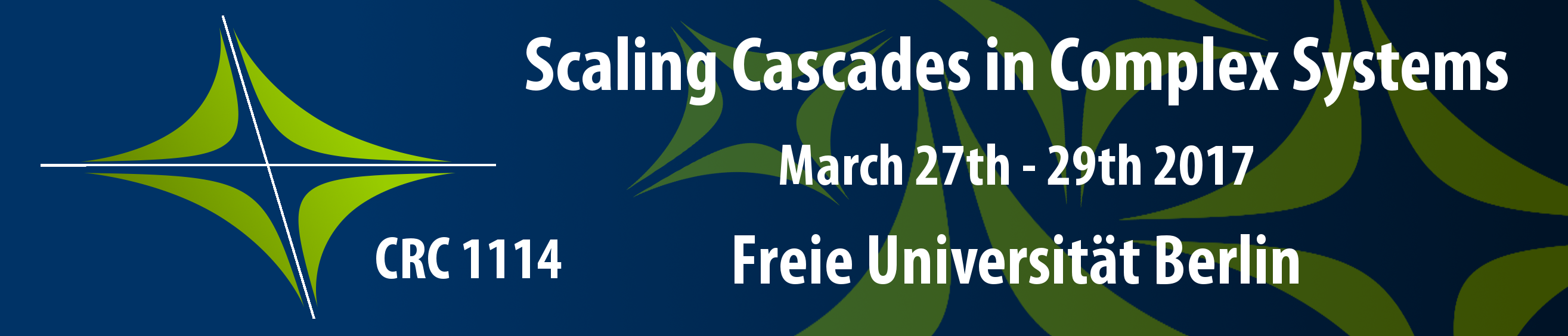### Keynote speakers in alphabetical order:

Carsten Eden - Universität Hamburg
Theoretical Oceanography

Prof. Eden’s main research interests are in understanding the large-scale circulation of the ocean, the effect and parameterising of (sub-) mesoscale variability as well as modelling and quantification of biogeochemical cycles in the ocean.

Gary Froyland - University of New South Wales
Mathematical Sciences - Dynamical Systems and Optimization

Prof. Froyland's dynamical systems research focusses on the interplay of probability and geometry in nonlinear and chaotic dynamical systems, and uses tools from ergodic theory.
His optimization research is focussed on decision making in large and complicated systems, sometimes in the presence of uncertain information. His research concerns new modelling approaches in mathematical programming, integer programming, and stochastic programming.

Taras Gerya - ETH Zürich
Earth Sciences

Prof. Gerya’s research focuses on plate tectonics, geodynamics, planetary processes as well as numerical geodynamic modelling.

Rupert Klein - Freie Universität Berlin
Mathematics and Computer Sciences

As speaker of CRC 1114, Prof. Klein oversees its various projects. In his research group "Geophysical Fluid Dynamics", his aim is to breach the gap between today's climate research computer simulations and established standards of applied mathematical modelling and numerical analysis.

Applied Mathematics

Prof. Michael Dellnitz's strength is the development of efficient algorithms for the numerical treatment of Dynamical Systems and  Multiobjective Optimization Problems. Research activities concentrate on both theoretical aspects of these algorithms and their numerical realization.

Mária Lukácová-Medvidová ­- Johannes Gutenberg-Universität Mainz
Numerical Mathematics

Prof. Lukácová-Medvidová’s research focuses on mathematical modelling and numerical simulations of complex flows. She deals with multidimensional hyperbolic conservation laws and multiscale problems arising in soft matters. She made contributions to the analysis and numerics of non-Newtonian, polymeric flows and fluid-structure interaction problems.Recently, she is investigating cancer invasion models both from the analytical as well as numerical point of view.

- Eindhoven University of Technology
Mathematics and Computer Science

Prof. Peletier’s research interest focuses on differential equations and their applications to real-world systems. His work spans the spectrum from applied to fundamental, with mathematical contributions to biology, geology, chemistry, pattern formation, solid mechanics, fluid dynamics, and contributions to the theory of variational calculus and differential equations.

- University of Bath
Mathematical Sciences - Numerical Analysis

Prof. Scheichl’s interests are in the design and analysis of efficient and robust parallel numerical methods for engineering and physical problems with heterogeneous material properties that vary over multiple scales. This is typical in energy and environmental applications, but also in material science and manufacturing.